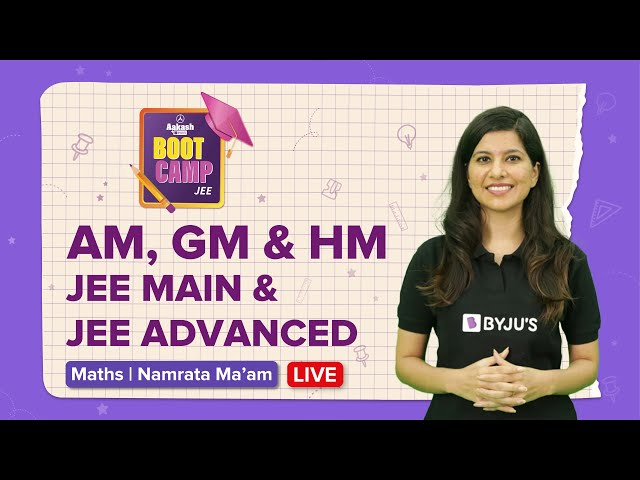Win up to 100% scholarship on Aakash BYJU'S JEE/NEET courses with ABNAT Win up to 100% scholarship on Aakash BYJU'S JEE/NEET courses with ABNAT

# Arithmetic Mean IIT JEE Study Material

If p, q, and r are in arithmetic progression (A.P.), then the middle term (q) is called the arithmetic mean (A.M.) of the other two terms (p and r). Therefore, the arithmetic mean between two numbers, p and r, is given by

$$\begin{array}{l}\mathbf{AM (q) = \frac{p\;+\;r}{2}}\end{array}$$

If p and q be any two given numbers, such that p, K1, K2, K3, K4,…, Kn, q are in arithmetic progression, then K1, K2, K3, K4,…, Kn are ‘n’ arithmetic means between p and q, such that

The first term = p and the (n + 2)th term = q

i.e., q = p + [ (n + 2) – 1] d = p + (n +1)d

Or,

$$\begin{array}{l}\mathbf{d = \frac{q\;-\;p}{n\;+\;1}}\end{array}$$

Therefore,

$$\begin{array}{l}\mathbf{K_{1}\;=\;p\;+\;\frac{q\;-\;p}{n\;+\;1}}\end{array}$$

Similarly,

$$\begin{array}{l}\mathbf{K_{2}\;=\;p\;+\;\frac{2\;(q\;-\;p)}{n\;+\;1}}\end{array}$$

Therefore,

$$\begin{array}{l}\mathbf{K_{n}=p+\frac{n\;(q\;-\;p)}{n\;+\;1}}\end{array}$$

Also, the sum of the ‘n’ arithmetic means inserted between p and q is equal to the ‘n’ times the single arithmetic mean between p and q.

$$\begin{array}{l}i.e.\ \mathbf{\sum_{r\;=\;1}^{n}\;K_{r}\;=\;n\;K}\end{array}$$
[where K = arithmetic mean between p and q]

## Arithmetic Mean of the mth Power

Let p1, p2, p3, p4,…, pn be n positive real numbers, and let m be a real number.

Case 1: m  (0, 1)

$$\begin{array}{l}\mathbf{\frac{p_{1}^{m}\;+\;p_{2}^{m}\;+\;p_{3}^{m}\;+\;.\;.\;.\;+\;p_{1}^{m}}{n}\;<\;\left ( \frac{p_{1}\;+\;p_{2}\;+\;p_{3}\;+\;.\;.\;.\;+\;p_{n}}{n} \right )^{m}}\end{array}$$

Case 2: m  {0, 1}

$$\begin{array}{l}\mathbf{\frac{p_{1}^{m}\;+\;p_{2}^{m}\;+\;p_{3}^{m}\;+\;.\;.\;.\;+\;p_{1}^{m}}{n}\;=\;\left ( \frac{p_{1}\;+\;p_{2}\;+\;p_{3}\;+\;.\;.\;.\;+\;p_{n}}{n} \right )^{m}}\end{array}$$

Case 3: m  R [0, 1]

$$\begin{array}{l}\mathbf{\frac{p_{1}^{m}\;+\;p_{2}^{m}\;+\;p_{3}^{m}\;+\;.\;.\;.\;+\;p_{1}^{m}}{n}\;>\;\left ( \frac{p_{1}\;+\;p_{2}\;+\;p_{3}\;+\;.\;.\;.\;+\;p_{n}}{n} \right )^{m}}\end{array}$$

## Relationship between Arithmetic, Geometric and Harmonic Mean

1. If A, H, & G be the arithmetic, harmonic and geometric mean of numbers p and q. Then,

$$\begin{array}{l}G^{2} = AH\end{array}$$

2. If A and G are A.M. and G.M. between two numbers a and b, then the quadratic equation whose roots are a and b can be written as

$$\begin{array}{l}x^{2} – 2Ax + G^{2} = 0\end{array}$$

3. In terms of inequalities: Arithmetic Mean ≥ Geometric Mean ≥ Harmonic Mean.

Arithmetic Mean IIT JEE Problems

Example 1: If a, b, and c are in arithmetic progression, then find the value of (a + 2b – c) (2b + c – a) (c + a – b).

Solution:

Since a, b, and c are in arithmetic progression, therefore,

$$\begin{array}{l}\mathbf{b\;=\;\frac{a\;+\;c}{2}}\end{array}$$

From the above equation, 2b – c = a, 2b – a = c, and a + c = 2b

Therefore, (a + 2b – c) (2b + c – a) (c + a – b) = (2a) (2c) (b) = 4abc.

Example 2: An even number of arithmetic means are inserted between 2 numbers, p and q, whose sum is 13/6. Find the total number of arithmetic means, if the sum of these arithmetic means exceeds their number by 1.

Solution:

Let the total number of arithmetic means inserted between two numbers, p and q, be 2n.

Since the sum of n AMs inserted between two numbers is equal to the n times, the single AM between those two numbers and the sum of arithmetic means = 2n + 1 (Given)

Therefore,

$$\begin{array}{l}\mathbf{2n\;\left [\; \frac{p\;+\;q}{2}\; \right ]\;=\;2n\;+\;1}\end{array}$$

i.e.

$$\begin{array}{l}\mathbf{n\;\left [\; \frac{13}{6}\; \right ]\;=\;2n\;+\;1}\end{array}$$

i.e., n = 6

so 2n = 12

Therefore, there are a total of 12 arithmetic means between the two numbers p and q.

Example 3: Find the value of ‘n’, if a total of n arithmetic means are inserted between 20 and 80, such that the ratio of 1st mean to the last mean is 1 : 3.

Solution:

The given sequence can be written as 20, K1, K2, K3, K4,…, Kn, 80.

Here, the 1st term (a) = 20 and the (n + 2)th term = 80

i.e., 80 = 20 + [ (n + 2) – 1] d

Or,

$$\begin{array}{l}\mathbf{d = \frac{60}{n\;+\;1}}\end{array}$$

Now, the first term of A.M. is given by,

$$\begin{array}{l}\mathbf{a + d = 20 + \frac{60}{n\;+\;1}}=\mathbf{\frac{20n\;+\;80}{n\;+\;1}}\end{array}$$

And the last term of A.M. is given by,

$$\begin{array}{l}\mathbf{a + nd = 20 +\frac{60\; \times \;n}{n\;+\;1}}=\mathbf{\frac{80\;n\;+\;20}{n\;+\;1}}\end{array}$$

According to the given condition,

$$\begin{array}{l}\mathbf{\frac{20n\;+\;80}{n\;+\;1}\;:\;\frac{80\;n\;+\;20}{n\;+\;1}\;=\;1\;:\;3}\end{array}$$

Or,

$$\begin{array}{l}\mathbf{\frac{20n\;+\;80}{n\;+\;1}\; \times \;\frac{n\;+\;1}{80\;n\;+\;20}\;=\;\frac{1}{3}}\end{array}$$

Or,

$$\begin{array}{l}\mathbf{\frac{n\;+\;4}{4n\;+\;1}\;=\;\frac{1}{3}}\end{array}$$

Therefore, n = 11

### AM, GM and HMTest Your Knowledge On Arithmetic Mean For Iit Jee!